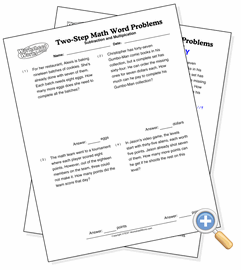# Two-Step Word Problems

## Subtraction, then MultiplicationSolve word problems that require two separate operations

Two-step word problems have three numbers which must be operated on separately, and in the right order. They take much more understanding of the problem than simple word problems because they provide the information in a less structured form. For these problems, finding the answer involves subtracting one number from another, then multiplying the difference by another number.